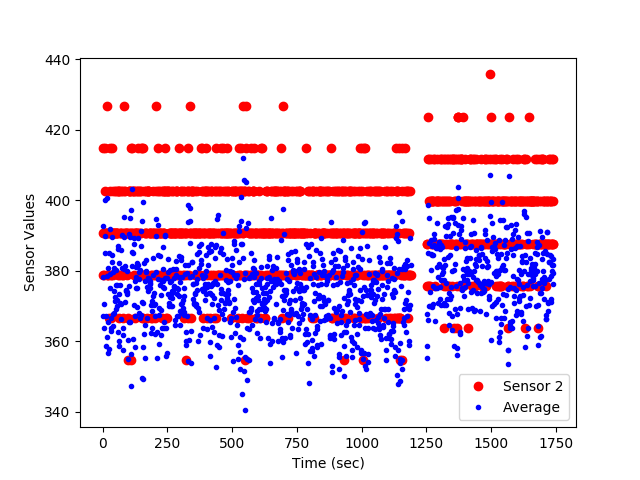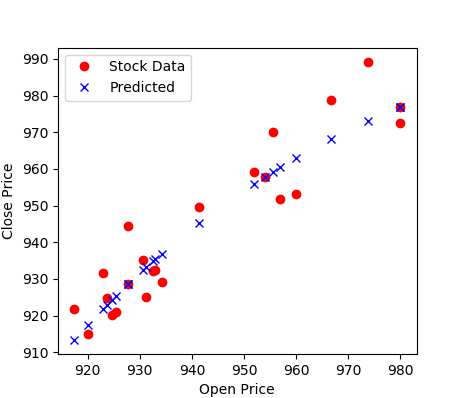### Data Analysis with Python

A common task for scientists and engineers is to analyze data from an external source that may be in a text or comma separated value (CSV) format. By importing the data into Python, data analysis such as statistics, trending, or calculations can be made to synthesize the information into relevant and actionable information. Tutorials below demonstrate how to import data (including online data), perform a basic analysis, trend the results, and export the results to another text file. Two examples are provided with Numpy and Pandas. Script files of the Python source code with sample data are below.

#### Import Data and Analyze with NumpyPython Data Analysis Example Source Code (Numpy)# Numpy (data import, manipulation, export)
import numpy as np
# Matplotlib (create trends)
import matplotlib.pyplot as plt

data_file = np.genfromtxt('data_file.txt', delimiter=',')

# create time vector from imported data (starts from index 0)
time = data_file[:,0]
# parse good sensor data from imported data
sensors = data_file[:,1:5]

# display the first 6 sensor rows
print(sensors[0:6])

# adjust time to start at zero by subtracting the
#  first element in the time vector (index = 0)
time = time - time

# calculate the average of the sensor readings
avg = np.mean(sensors,1) # over the 2nd dimension

# export data
# stack time and avg as column vectors
my_data = np.vstack((time,sensors.T,avg))
# transpose data
my_data = my_data.T
# save text file with comma delimiter
np.savetxt('export_from_python.txt',my_data,delimiter=',')

# generate a figure
plt.figure(1)
plt.plot(time/60.0,sensors[:,1],'ro')
plt.plot(time/60.0,avg,'b.')
# add text labels to the plot
plt.legend(['Sensor 2','Average Sensors 1-4'])
plt.xlabel('Time (min)')
plt.ylabel('Sensor Values')
# save the figure as a PNG file
plt.savefig('my_Python_plot.png')
# show the figure on the screen (pauses execution until closed)
plt.show()

#### Import Data and Analyze with PandasData File with HeadersPython Data Analysis Example Source Code (Pandas)

# import Numpy, Pandas, and Matplotlib
import numpy as np
import pandas as pd
import matplotlib.pyplot as plt

# create time vector from imported data
time = data_file['time']
# parse good sensor data from imported data
sensors = data_file.loc[:, 's1':'s4']

# display the first 6 sensor rows
print(sensors[0:6])

# adjust time to start at zero by subtracting the
#  first element in the time vector (index = 0)
time = time - time

# calculate the average of the sensor readings
avg = np.mean(sensors,1) # over the 2nd dimension

# export data
my_data = [time, sensors, avg]
result = pd.concat(my_data,axis=1)
result.columns.values[-1] = 'avg'

result.to_csv('result.csv')
#result.to_excel('result.xlsx')
result.to_html('result.htm')
result.to_clipboard()

# generate a figure
plt.figure(1)
plt.plot(time,sensors['s1'],'r-')
plt.plot(time,avg,'b.')
# add text labels to the plot
plt.legend(['Sensor 2','Average'])
plt.xlabel('Time (sec)')
plt.ylabel('Sensor Values')
# save the figure as a PNG file
plt.savefig('my_Python_plot.png')
# show the figure on the screen
plt.show()

#### Import Online Data and Analyze

Below is an example of pulling data from an Internet source, such as financial information about a stock. The example shows how to request, parse, and display the financial data.Google Stock Data File

import pandas as pd
import matplotlib.pyplot as plt

# stock ticker symbol

# import data with pandas
print(data['Close'][0:5])
print('min: '+str(min(data['Close'][0:20])))
print('max: '+str(max(data['Close'][0:20])))

# plot data with pyplot
plt.figure()
plt.plot(data['Open'][0:20])
plt.plot(data['Close'][0:20])
plt.xlabel('days ago')
plt.ylabel('price')
plt.show()

Once the data is imported, it can be analyzed with many different tools such as machine learning algorithms. Below is an example of using the data for analysis of correlation between open and close price of Google publicly traded shares.from gekko import GEKKO
import numpy as np
import matplotlib.pyplot as plt
import pandas as pd

# import data with pandas
print(data['Close'][0:5])
print('min: '+str(min(data['Close'][0:20])))
print('max: '+str(max(data['Close'][0:20])))

# GEKKO model
m = GEKKO()

# input data
x = m.Param(value=np.array(data['Open']))

# parameters to optimize
a = m.FV()
a.STATUS=1
b = m.FV()
b.STATUS=1
c = m.FV()
c.STATUS=1

# variables
y = m.CV(value=np.array(data['Close']))
y.FSTATUS=1

# regression equation
m.Equation(y==b*m.exp(a*x)+c)

# regression mode
m.options.IMODE = 2

# optimize
m.options.solver = 1
m.solve(disp=True)

# print parameters
print('Optimized, a = ' + str(a.value))
print('Optimized, b = ' + str(b.value))
print('Optimized, c = ' + str(c.value))

# plot data
plt.figure()
plt.plot(data['Open'],data['Close'],'ro',label='Stock Data')
plt.plot(x.value,y.value,'bx',label='Predicted')
plt.xlabel('Open Price')
plt.ylabel('Close Price')
plt.legend()
plt.show()

This tutorial can also be completed with Excel and Matlab. Click on the appropriate link for additional information.